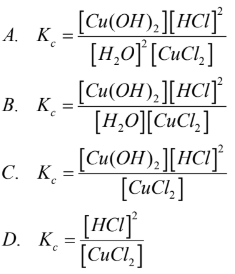# Problem: The equilibrium constant associated with the reactionCu(OH)2(s) + 2HCl(aq) ⇌ 2H2O(l) + CuCl2(aq) is...E. None of the above

###### FREE Expert Solution

We're being asked to write the equilibrium constant associated with the reaction:

Cu(OH)2(s) + 2 HCl(aq) ⇌ 2 H2O(l) + CuCl2(aq)

Kc is the equilibrium expression of the concentrations in the reaction. The general form of an equilibrium expression is:

$\overline{){{\mathbf{K}}}_{{\mathbf{c}}}{\mathbf{=}}\frac{\mathbf{products}}{\mathbf{reactants}}}$

Given reaction:

87% (65 ratings)###### Problem Details

The equilibrium constant associated with the reaction

Cu(OH)2(s) + 2HCl(aq) ⇌ 2H2O(l) + CuCl2(aq) is...E. None of the above